## Challenge Summary#

Lost in Your Eyes is a reverse engineering challenge in DiceCTF 2021 with ten solves (334 points).

Your eyes are like a maze, and I hate mazes, so help me escape.

We are given a binary which takes an input and outputs either :) or :(. If you win a smiley face on the remote server, you are additionally given the flag.

## Solution#

This challenge is solved by harrier in collaboration with Mystiz. The solution is written in the prespective of harrier.

### Part I: Reversing the virtual machine#

Since this is a reverse question with a binary, I decided to open it with IDA. This is a simple VM-type binary, with the instruction code stored directly inside.

It looked so simple to me at the beginning, and I wonder why no one has solved it? After a bit of reversing and dynamically debugging, I know I was wrong.

The code of the binary itself is simple. It is a simple VM with the below variables

• Eight one-byte state registers ($R_0, R_1, …, R_7$),
• a two-byte instruction pointer ($p$),
• an one-byte direction register ($d=1, 2, 3, 4$) deciding how the instruction pointer move,
• an one-byte select register ($k$) deciding the index of register for subsequent operations, and
• 65536 one-byte memory ($M_0, M_1, …, M_{65535}$) storing the instructions and data.

Moreover, each byte in the memory represents an instruction, and the instruction set is defined below.

Value Operation
0 do nothing
1 - 8 $k \leftarrow 0$, …, $k \leftarrow 7$
9 - 16 $R_k \leftarrow R_0$, …, $R_k \leftarrow R_7$
17 $R_k \leftarrow R_k + 1$
18 $R_k \leftarrow R_k - 1$
19 $R_k \leftarrow R_6 + R_7$
20 $R_k \leftarrow R_6 - R_7$
21 $R_k \leftarrow R_6 \times R_7$
22 $R_k \leftarrow \text{floor}(R_6 / R_7)$
23 $R_k \leftarrow R_6\ \text{mod}\ R_7$
24 $R_k \leftarrow \text{~}R_k$
25 $R_k \leftarrow -R_k$
26 $R_k \leftarrow R_6\ \text{and}\ R_7$
27 $R_k \leftarrow R_6\ \text{or}\ R_7$
28 $R_k \leftarrow R_6\ \text{xor}\ R_7$
29 $R_k \leftarrow R_6 == R_7$
30 $R_k \leftarrow R_6 < R_7$
31 $256R_0+R_1 \leftarrow p, R_2 \leftarrow d$
32 keycheck
33 $R_k \leftarrow M_{256R_0 + R_1}$
34 $M_{256R_0 + R_1} \leftarrow R_k$
35 $p \leftarrow 256R_0 + R_1, d \leftarrow R_2$
36 $R_k \leftarrow \text{input}$
37 $\text{print}\ R_k$
38 - 41 $d \leftarrow 1$, …, $d \leftarrow 4$

Keycheck is the only curious instruction, where I don’t really know why it even exists. While I was reversing, I am only able to realize it is used perform some fatal checks.

### Part II: Building an emulator#

As the VM code is so simple, I decided to write an emulator in Python for ease of testing. It is not hard to write an emulation given that I have fully reversed the VM.

Since the instruction code is embedded in the binary, I used gef to extract the code part, and while in emulation I translate the code to human (me) readable instruction for further reverse. I thought I could get the flag by just reading and parsing the translated code, and figuring out the whole logic. I was wrong and it was far more complicated than that.

With the emulator I was able to produce some code trace with various input, here’s some debug log I generated, with state of the VM and instruction it ran:

Current state: [0, 46, 1, 22, 41, 35, 23, 0] 6 50 / 16 0
50/16 REGSEL = 6
Current state: [0, 46, 1, 22, 41, 35, 24, 0] 6 49 / 16 0
Current state: [0, 46, 1, 22, 41, 35, 24, 0] 7 48 / 16 0
48/16 REGSEL = 7
Current state: [0, 46, 1, 22, 41, 35, 24, 0] 7 47 / 16 0
47/16 REG = REG == REG
Current state: [0, 46, 1, 22, 41, 35, 24, 0] 7 46 / 16 0
46/16 KEYCHECK REG OK
Current state: [0, 46, 1, 22, 41, 35, 24, 0] 7 45 / 16 3
45/16 SETMODE 3
Current state: [0, 46, 1, 22, 41, 35, 24, 0] 7 45 / 15 2
45/15 SETMODE 2
Current state: [0, 46, 1, 22, 41, 35, 24, 0] 7 46 / 15 2
47/15 REG = REG


a/b is the notation I used to express instruction pointers, hereby $p = 256a + b$.

I yield thousands of debug lines like the one above, and I tried to reverse the input process logic, and I realize there is a loop to detect what is my input, and it loop through to see whether the input matched those in the accepted characters.

How the characters are matched? Only here I realize the meaning of the keycheck operation. It is simply an if: If the condition holds, the instruction pointer will move two steps forward. Otherwise it will move only one.

Also, I was able to generate a list of possible inputs for the first byte, but then I was stuck and didn’t know how to do.

### Part III: Visualizing the inner program#

This part is written in the prespective of Mystiz.

I decided to join harrier on this challenge at some point of time. At that moment, he have reversed all of the VM and part of the inner program. I want to know what proportation of the inner program he has reversed.

At first, I tried to use graphviz for visualization. The results are pretty dull, and most nodes have both the in-degrees and out-degrees being one.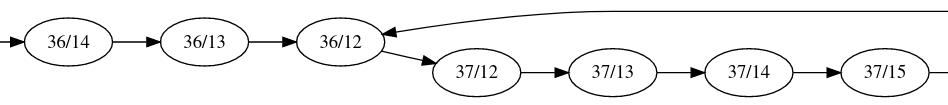This is not surprising, since the nodes have out-degrees larger than one only if they are branches. However, one interesting point from this is, in most of the time, if x1/y1 transits to x2/y2, then $|x_1-x_2|+|y_1+y_2|=1$… Sounds like they are transited to adjacent grids in each instruction. I recalled some esolangs like Piet and Befunge, where the instruction pointers are actually 2D-coordinates. Although they are not equal, I had an idea to transform the whole thing into a two-dimensional grid. Well, maybe I should use HTML tables to visualize this. Since harrier is tracing the instructions executed, I can integrate it and generate a heatmap to check what is being covered.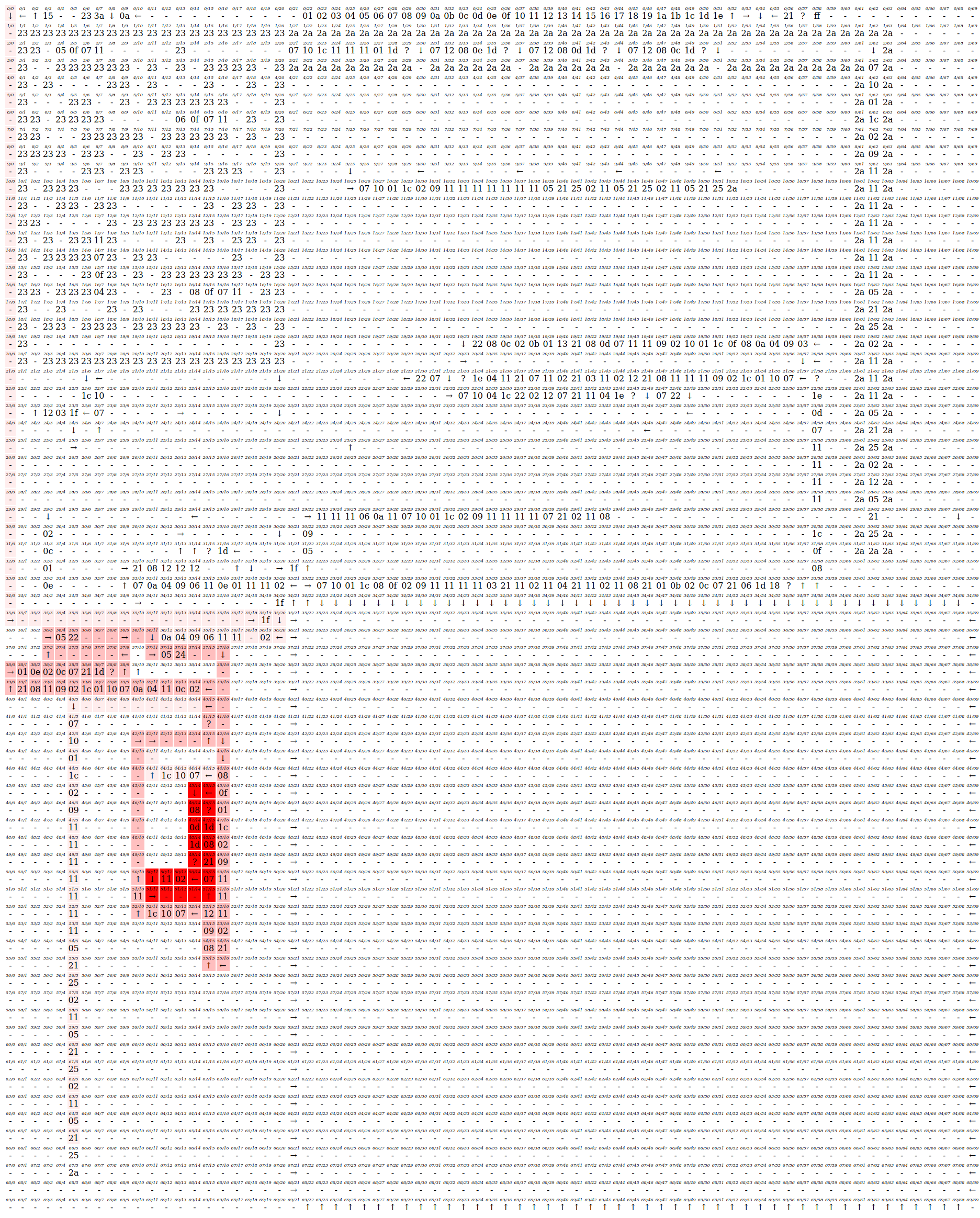Wow, this is pretty impressive. Now we can see that only a small proportion of the code in the inner program is visited. One thing that caught our attention is the maze on the top-left corner. Well, it seemed impossible for us to traverse inside the maze, since there are no arrows and we are very likely unable to turn without arrows.

We are able to identify a few zones inside the internal program:

• Request for input
• Loop structure
• The failing and the winning gadgets
• Maze

### Part IV: Generating random ideas#

While testing with random inputs, it seemed that the input will be filling in 35/22, 35/23 and etc.

My first idea is to see whether if we can overwrite 35/1 by overflowing the input buffer. I was expecting that the original code can be corrupted by writing up to 35/255, then it will be wrapped back to 35/0 and 35/1. Turns out this is not possible since only the bottom right zone, with dimensions $47\times34$, could be overwritten, filling line by line. The excessive input will simply be ignored.

From the visualization, we can see that there are several print gadgets. For example, there is a failing gadget (which prints :() on the bottom left input part that will be traversed when a character outside the character set is given. There is also one on the upper middle of the map and one in the top right. Mystiz has identified that the former one has the same instructions as the one on the bottom left, so it would also be a failing gadget. The objective is simple: To make the instruction pointer end up on the top-right part, on the winning gadget.

To achieve this, one idea we had is to find ways to jump to the winning gadget directly. We found that the instruction pointer will be moving into the payload area when we are given a sufficiently long input. Therefore we can actually execute the payloads we sent.

Knowing that, we tried to use the set address instruction (byte 0x23) to jump to the win widget. Unfortunately this does not work, since 0x23 is not allowed as an input.

We then come up an idea to try to use the conditional keycheck operation on the side of the square to escape out of the box. However keycheck does not allow an arrow pointing to itself. This does not work as well.

It seems that the only possible way is to navigate in the maze properly to get to the win gadget. But how is it possible if the maze do not have arrows?

Well, there is an untouched part of the program - the arrow-putting gadget. With $R_4 < 4$, it can be used to put arrows on to the maze with the set register instruction (byte 0x21). We reversed it and and expected that it behaves as the following Python code snippet:

for x in range(1, 21):
for y in range(1, 21):
# i.e., the walls.
if M[256*x + y] == 0x23: continue
if R < 4:
M[256 * x + y] = 0x26 + R
# 0x26 0x27 0x28 0x29
#   ↑    →    ↓    ←


Our objective is updated once again. Now the goal is to craft a payload to put appropriate arrows to let us navigate through the maze.

But wait, there is a check after the maze. Basically, there are some instructions in the maze to write $R_3, R_4, R_5$ and $R_7$. All we need to do is to walk through the maze in a specific order to pass the check.

So we finally have a clear target: design a route for the maze to pass through all the checks and get to the winning gadget.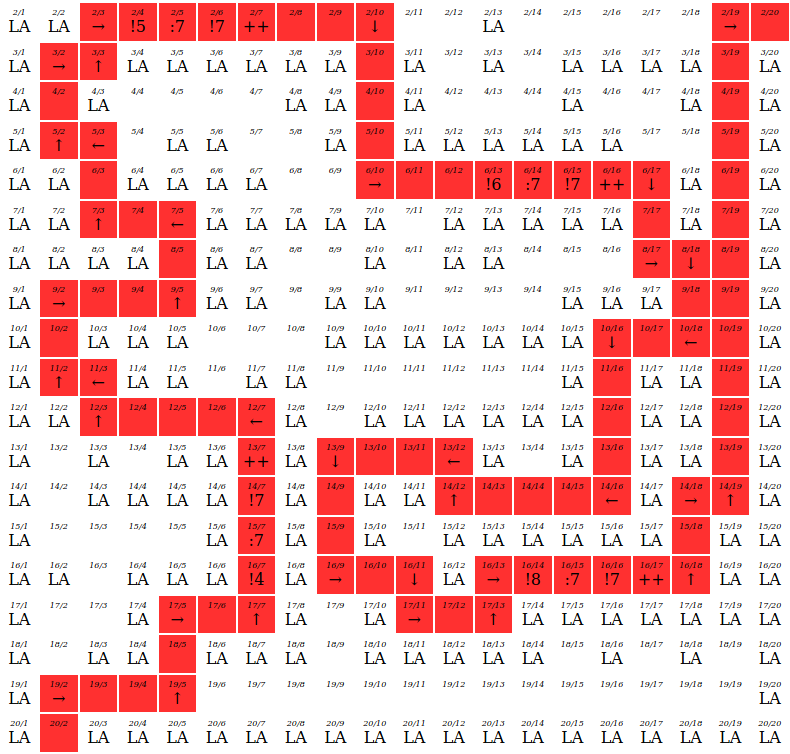### Part V: Crafting a smiley face#

In each iteration, I can control 8 registers, initially with $R_0 = 0, R_1 = 5, R_6 = x$ and $R_7 = y$. Initially, I copied the coordinates by $R_2 \leftarrow x$ and $R_3 \leftarrow y$ for further operations. Moreover, $R_0$ and $R_1$ can be used to build up to an arbitrary number in a few instructions.

The idea is to encode few arrows into a vertical block.

However, the size of our input is pretty small. We need to make use of some techniques to save space. For instance, we can encode few arrows into one condition (Instead of checking x == x0 and y == y0, we can use y > y0, y mod 6 or even x == y as long as it traverses to the goal).

Writing the payload is tiring without some auxiliary tools, so I used spreadsheet to write the instructions and wrote a simple CSV parser to convert those instruction to the input payload. After some unknown hours of manual work, I have eventually crafted the payload. This is how it looks in spreadsheet. 🙈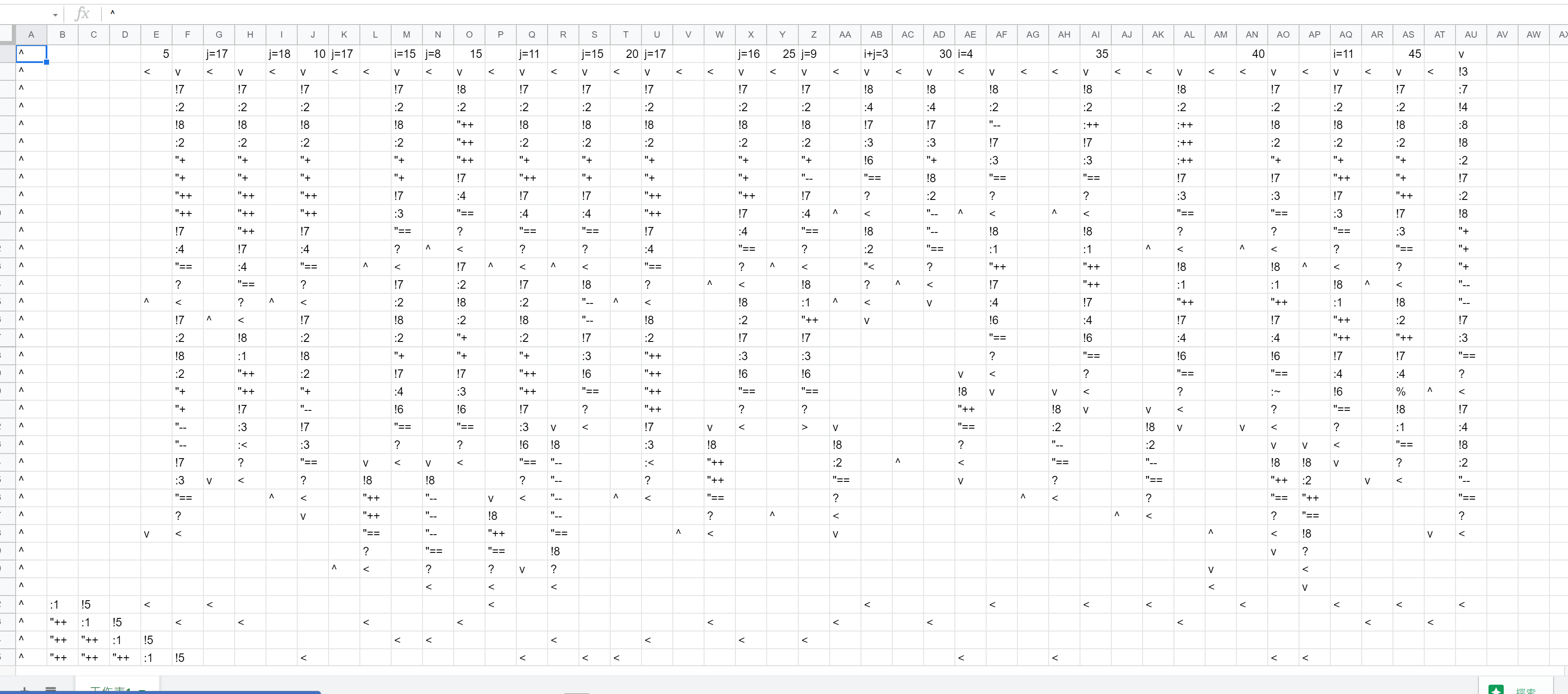It is then converted as a payload to the binary. This is the emulated result that eventually returns a smiley face.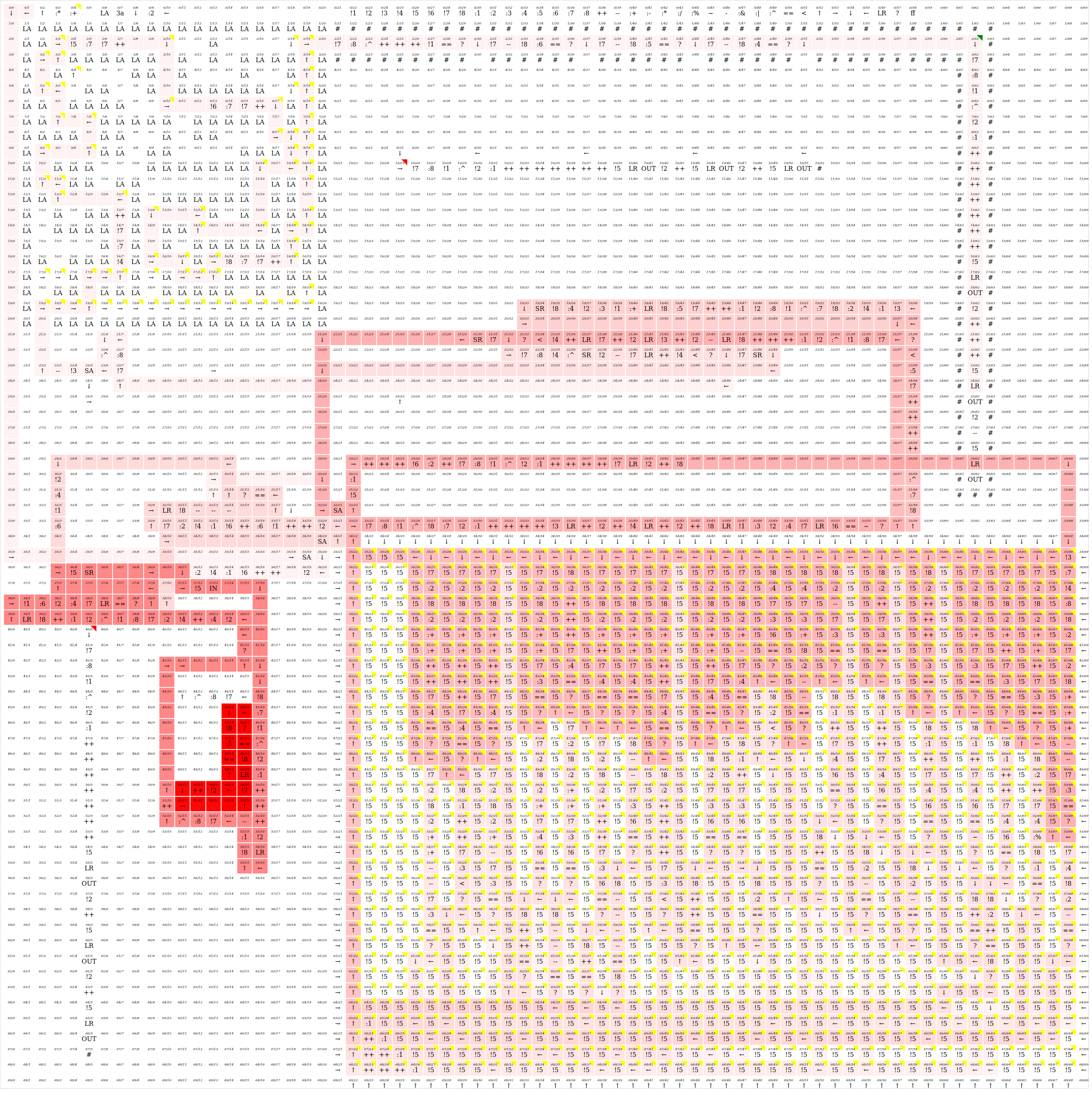### Part VI: Final thoughts#

It was really a fun reverse challenge, while being easy to begin yet challenging to finish. Every part of the challenge is well-designed. The maze, the constraints, and even the instructions that making this challenge to work. That was an exciting and mind-blowing experience to explore and dig into rabbit holes of the challenge bit by bit.

Thanks to Mystiz for the great insights and the visualization, I think having the visualization itself is already having half of the challenge solved. 😀